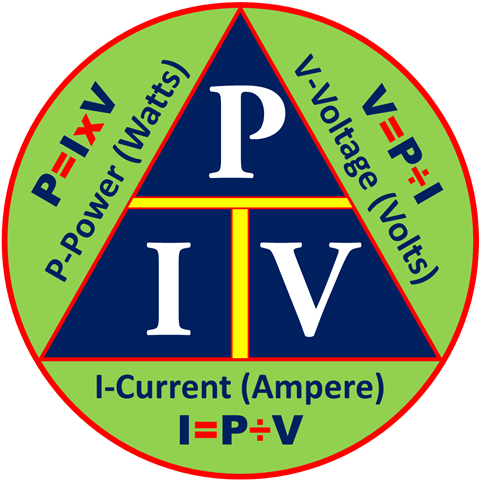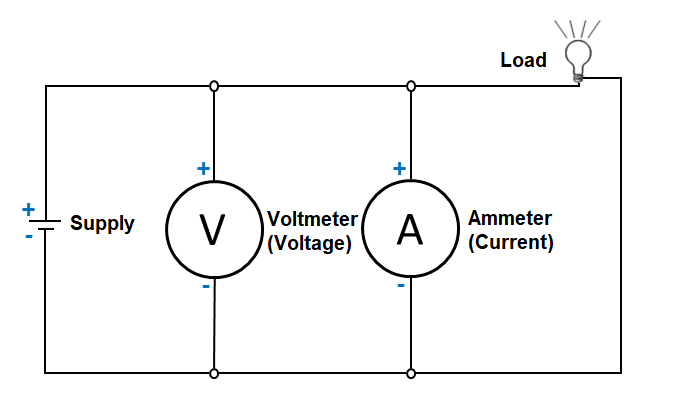## The Electricity Formula Wheel

What is the Electricity Formula Wheel? This is sometimes called Resistance Formula Wheel or simply Formula Wheel. But for the purpose of specificity, we will use the “Electricity Formula Wheel”. It is used as reference to solve for the unknown value in an electrical circuit to help builders and maintenance teams design, install, repair and properly implement electrical systems and applications. This formula covers the four major areas involved in a circuit, namely, Voltage, Current, Resistance and Power.

Resistance in relation to Voltage and Current has been discussed in the two previous lessons, extracting the needed formulas and converting them into a Triangle Formula. In this lesson and in the next three, we will discuss how to use the same principle in calculating the relationship between Power, Current and Voltage.As in Ohm’s Law, the same principle applies in calculating the Power, Voltage and Current where the use of the formulas can only give the correct answer when the correct values are used. So always remember the following four rules:

– Current is always expressed in Amperes or amps
– Voltage is always expressed in Volts
– Resistance is always expressed in Ohms
– Power is always expressed in Watts

With these rules, the decimal equivalent of figures that are less than 1 should be converted. For example, if the circuit’s voltage is 10 and the current is 200 mA (200 milliamp), the decimal equivalent of the 200 mA, which is 0.2 Amp, should be used  in the calculation.

In the next three lessons, we will extract, or individualize, the formulas for Power, Voltage and Current into their triangle formula versions. Then we will use these to solve for the unknown values.

The Power, Current, Voltage Formula TriangleHow do we use the formula triangle?

When we use the triangles to solve the unknown values, the given equivalent figure on the apex of the triangle is divided by either of the values at the base of the the triangle, whichever is the given meter reading or electronic value. To find the value of the apex, a given value at either side of the base of the triangle is multiplied by the other base value.

Let’s start now. You may proceed to the next lesson to practice using this.

Summary: The electricity formula wheel is a combination or compilation of the different triangle formulas that provides solutions to solving or calculating the resistance, current, voltage and power consumption of an electrical circuit.

When we use the triangles to solve the unknown values, the given equivalent figure on the apex of the triangle is divided by either of the values at the base of the the triangle, whichever is the given meter reading or electronic value. A given value at either side of the base of the triangle is multiplied by the other base value, and vice versa.

Posted in